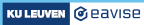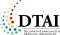3.6 Counting

### Counting

Existential quantifiers ("`?`") give us a rudimentary way of counting how many values satisfy a certain property. As we already know, the following formula expressed that at least one node is coloured green.

```?x in Node: colourOf(x) = green.
```

By then using two quantifiers, we can express that at least two nodes are green:

```?x, y in Node: colourOf(x) = green & colourOf(y) = green & x ~= y.
```

We can carry on like this, e.g.:

```?x, y, z in Node: colourOf(x) = green & colourOf(y) = green
& colourOf(z) = green & x ~= y & z ~= y & x ~= z.
```

(Note that this formula requires three inequalities because, in contrast to `=`, the `~=` operator is not transitive, i.e., it is not because `x ~= y` and `y ~= z` that also necessarily `x ~= z`.)

We can also combine these kind of formulas to say that, for instance, precisely 1 node is green.

```(?x in Node: colourOf(x) = green)
& ~(?x, y in Node: colourOf(x) = green & colourOf(y) = green & x ~= y).
```

(Here, the first part of the formula states that at least one node is green, while the second part specifies that there cannot be more than two green nodes. Thus, exactly one must be green!)

Obviously, this way of counting is not very convenient. For this reason, there exists a useful shortcut called the cardinality aggregate ("`#{ ... }`"). For instance, the following expression refers to the number of green nodes:

```#{ x in Node: green(x) }
```

We can then use such a term to say that, e.g., there are precisely two such green nodes:

```#{ x in Node: green(x) } = 2.
```

### Exercise

Add an additional constraint to the node colouring problem, to specify that more nodes must be coloured red than green.

###### Made with love (and Hugo) by Joost Vennekens and Simon Vandevelde. :-)